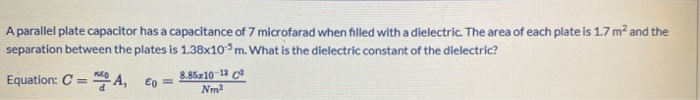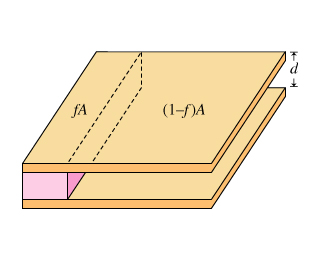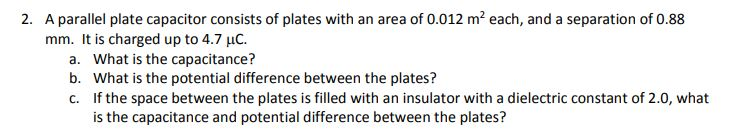Question

# A parallel-plate capacitor is filled with a dielectric with K = 4. The plate area is 3 m2 and...

A parallel-plate capacitor is filled with a dielectric with K = 4. The plate area is 3 m2 and the plate separation is 2 mm. The capacitance of this capacitor is

a) 210 nF.
b) 18 nF.
c) 8 nF.
d) 13 nF.
e) 53 nF.

capacitance C = ε0 A/r , ε0 = 8.854x10-12 , A = 3 m^2 , r = 2 mm = 0.002 , k=4
e) 53 nF.

Given dataDielectric constant, K = 4Area of the plate is, A = 3 m2Plate seperation is, d = 2 mm= (2 mm) (10-3 m / 1mm)= 2 * 10-3 mSolution:The capciatance of the capacitor is,C= ε0KA / d= (8.854 * 10-12 F/m) (4) (3 m2) / (2 * 10-3 m )= 53.1 * 10-9 F˜ 53 nFOption (e) is correct

capacitance C = ε0 A/r , ε0 = 8.854x10-12 , A = 3 m^2 , r = 2 mm = 0.002 , k=4
e) 53 nF.

a) 210 nF.
a) 210 nF.

#### Earn Coins

Coins can be redeemed for fabulous gifts.

Similar Homework Help Questions
• ### A dielectric-filled parallel-plate capacitor has plate area A = 20.0 cm2 , plate separation d =...

A dielectric-filled parallel-plate capacitor has plate area A = 20.0 cm2 , plate separation d = 8.00 mm and dielectric constant k = 3.00. The capacitor is connected to a battery that creates a constant voltage V = 15.0 V . Throughout the problem, use ϵ0 = 8.85×10−12 C2/N⋅m2 A) Find the energy U1 of the dielectric-filled capacitor B) The dielectric plate is now slowly pulled out of the capacitor, which remains connected to the battery. Find the energy U2...

• ### A dielectric-filled parallel-plate capacitor has plate area A = 25.0 cm2 , plate separation d =...

A dielectric-filled parallel-plate capacitor has plate area A = 25.0 cm2 , plate separation d = 10.0 mm and dielectric constant k = 2.00. The capacitor is connected to a battery that creates a constant voltage V = 15.0 V . Throughout the problem, use ϵ0 = 8.85×10−12 C2/N⋅m2 1. Find the energy U1 of the dielectric-filled capacitor. 2. The dielectric plate is now slowly pulled out of the capacitor, which remains connected to the battery. Find the energy U2...

• ### A dielectric-filled parallel-plate capacitor has plate area A = 30.0 cm2 , plate separation d =...

A dielectric-filled parallel-plate capacitor has plate area A = 30.0 cm2 , plate separation d = 9.00 mm and dielectric constant k = 4.00. The capacitor is connected to a battery that creates a constant voltage V = 15.0 V . Throughout the problem, use ϵ0 = 8.85×10−12 C2/N⋅m2 . A. Find the energy U1 of the dielectric-filled capacitor. B.The dielectric plate is now slowly pulled out of the capacitor, which remains connected to the battery. Find the energy U2...

• ### A dielectric-filled parallel-plate capacitor has plate area A =15.0 cm2 , plate separation d =...

A dielectric-filled parallel-plate capacitor has plate area A = 15.0 cm2 , plate separation d = 10.0 mm and dielectric constant k = 2.00. The capacitor is connected to a battery that creates a constant voltage V = 15.0 V . Throughout the problem, use ϵ0 = 8.85×10−12 C2/N⋅m2 . (All answers in Joules)Part A.) Find the energy U1 of the dielectric-filled capacitor.Part B.) The dielectric plate is now slowly pulled out of the capacitor, which remains connected to the...

• ### A dielectric-filled parallel-plate capacitor has plate area A = 30.0 cm2 , plate separation d =...

A dielectric-filled parallel-plate capacitor has plate area A = 30.0 cm2 , plate separation d = 6.00 mm and dielectric constant k = 3.00. The capacitor is connected to a battery that creates a constant voltage V = 5.00 V . Throughout the problem, use ϵ0 = 8.85×10−12 C2/N⋅m2 a) The dielectric plate is now slowly pulled out of the capacitor, which remains connected to the battery. Find the energy U2 of the capacitor at the moment when the capacitor...

• ### A parallel plate capacitor has a capacitance of 7 microfarad when filled with a dielectric. The...A parallel plate capacitor has a capacitance of 7 microfarad when filled with a dielectric. The area of each plate is 1.7 m2 and the separation between the plates is 1.38x10m. What is the dielectric constant of the dielectric? Equation: C = A, 60 = 8.85z10-12 Nm2

• ### A parallel-plate capacitor has plates of area 3.55×10−4 m2 A) What plate separation is required if...

A parallel-plate capacitor has plates of area 3.55×10−4 m2 A) What plate separation is required if the capacitance is to be 1490 pF ? Assume that the space between the plates is filled with air? (Dielectric constant for air is 1.00059) B) What plate separation is required if the capacitance is to be 1490 pF ? Assume that the space between the plates is filled with paper. (Dielectric constant for paper is 3.7)

• ### Consider a parallel-plate capacitor that is partially filled with a dielectric of dielectric constant KConsider a parallel-plate capacitor that is partially filled with a dielectric of dielectric constant K. The dielectric has the same same height as theseparation of the plates of the capacitor butfills a fraction f of the area of the capacitor. The capacitance of thecapacitor whenthe dielectric is completely removed is C0.What is the capacitance C(f) of this capacitor as afunction of f? Express C(f) in terms of C0, f,and K.

• ### A parallel plate capacitor consists of plates with an area of 0.012 m2 each, and a...A parallel plate capacitor consists of plates with an area of 0.012 m2 each, and a separation of 0.88 mm. It is charged up to 4.7 uC. 2. a. b. c. What is the capacitance? What is the potential difference between the plates? If the space between the plates is filled with an insulator with a dielectric constant of 2.0, what is the capacitance and potential difference between the plates?

• ### A parallel plate capacitor is constructed with plate area of 0.40 m2 and a plate separation...

A parallel plate capacitor is constructed with plate area of 0.40 m2 and a plate separation of 0.10 mm. How much charge is stored on it when it is charged to a potential difference of 12 V? A parallel-plate capacitor is filled with air, and the plates are separated by 0.050 mm. If the capacitance is 17.3 pF, what is the plate area? A parallel plate capacitor is constructed with plate area of 0.0010 m2 and a plate separation of...